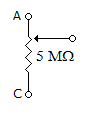# Electronics and Communication Engineering - Networks Analysis and Synthesis

1.

In an RL series circuit the inductance is 1H and current at an instant is 0.6 A. The energy stored in magnetic field is

 A. 0.36 J B. 0.18 J C. 0.09 J D. 0.03 J

Explanation:

Energy = 0.5 LI2 = 0.5 x 1 x 0.62 = 0.18 J.

2.

In a series ac circuit a voltage of 20 V at 25 Hz causes a current of 0.20 A, while the same voltage at 75 Hz causes a current of 0.12 A. The circuit consists of

 A. R and C in parallel B. R and C in series C. R and L in series D. either (a) or (b)

Explanation:

As f increases impedance increases and current decreases in RL circuit.

3.

Elements R, L, C are connected in parallel and excited by a dc current I. If iR, iL and iC are the currents through R, L, C respectively then at t = 0

 A. iR > iL > iC B. iL > iR > iC C. iC = 0 D. iC = I

Explanation:

Capacitor behaves as a short-circuit at t = 0.

4.

In a practical voltage source, the terminal voltage

 A. cannot be less than source voltage B. cannot be higher than source voltage C. is always less than source voltage D. is always equal to source voltage

Explanation:

A practical voltage source has some source resistance.

Because of voltage drop across source resistance, terminal voltage cannot be higher than source voltage.

If current is zero, terminal voltage and source voltage are equal.

5.

When slider of the potentiometer AB is in the position shown in figure, what resistance reading would be indicated by an ohmmeter connected between A and C?A. zero B. infinite C. can't say D. none of the above